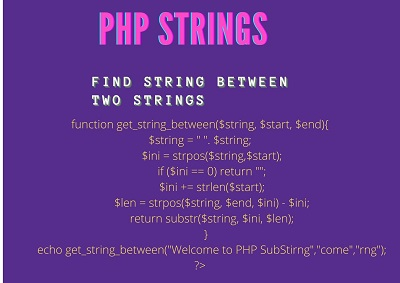# PHP String Parser | Find String Between String

Last updated Feb 11, 2021

In this post we will learn find string between string in PHP, before that we need to know

What is String?

Combination of characters or sequesnce of characters is called String. PHP Contains a data type as String.

The String in PHP contains build in functions like

str_contains — Determine if a string contains a given substring
str_ends_with — Checks if a string ends with a given substring
str_getcsv — Parse a CSV string into an array
str_ireplace — Case-insensitive version of str_replace
str_pad — Pad a string to a certain length with another string
str_repeat — Repeat a string
str_replace — Replace all occurrences of the search string with the replacement string

explode — Split a string by a string

Create a String in PHP

There is 4 ways to create a Stirng in PHP

• Creating Strings Using Single quotes
• Creating String Using Double quotes

• ## NowdocHere the function created to find the string between stirngs in php.

Look at the code

 Article Source: http://EzineArticles.com/3097571 ``` ```

Output

`to PHP SubSti`

In the above function we need to pass the three variables.

First one is the string where we need to check the in between string

second and third variable are the string that we need to check between

The functionality in the above is First, find the integer position of the the left side and then add that to the total length.

Next find the integer position of the right side data point starting at the integer position equal to the length of the left point and then subtract that by the total length of the left.

Related Topics

PHP MockTest Questions

The mechanics here are very simple. First, find the integer position of the the left point and then add that to the total length. Next find the integer position of the right data point starting at the integer position equal to the length of the left point and then subtract that by the total length of the left.

Article Source: http://EzineArticles.com/3097571

Article Source: http://EzineArticles.com/3097571
<?php function get_string_between(\$string, \$start, \$end){   \$string = ''. \$string; \$ini = strpos(\$string,\$start); if (\$ini == 0) return ""; \$ini += strlen(\$start); \$len = strpos(\$string, \$end, \$ini) - \$ini; return substr(\$string, \$ini, \$len); } echo get_string_between("Welcome to PHP SubStirng","come","rng"); ?>

Output

to PHP SubSti

In the above function we need to pass the three variables.

First one is the string where we need to check the in between string

second and third variable are the string that we need to check between

The functionality in the above is First, find the integer position of the the left side and then add that to the total length.

Next find the integer position of the right side data point starting at the integer position equal to the length of the left point and then subtract that by the total length of the left.

Related Topics

PHP MockTest Questions

The mechanics here are very simple. First, find the integer position of the the left point and then add that to the total length. Next find the integer position of the right data point starting at the integer position equal to the length of the left point and then subtract that by the total length of the left.

Article Source: http://EzineArticles.com/3097571

-->
 Article Contributed By :484 Views
Projects By category
Categories